# Sum if Across Multiple Sheets – Excel & Google Sheets

This tutorial will demonstrate how to use the SUMPRODUCT and SUMIFS Functions to sum data that meets certain criteria across multiple sheets in Excel and Google Sheets.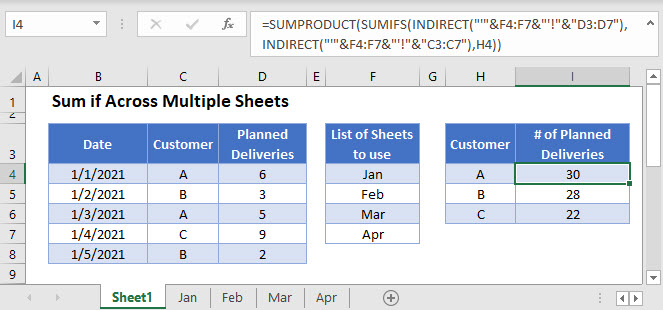## Regular Sum Across Multiple Sheets

Sometimes your data might span several worksheets in an Excel file. This is common for data that is collected periodically. Each sheet in a workbook might contain data for a set time period. We want a formula that sums data contained in two or more sheets.

The SUM Function allows you to easily sum data across multiple sheets using a 3D Reference:

``=SUM(Sheet1:Sheet2!A1)``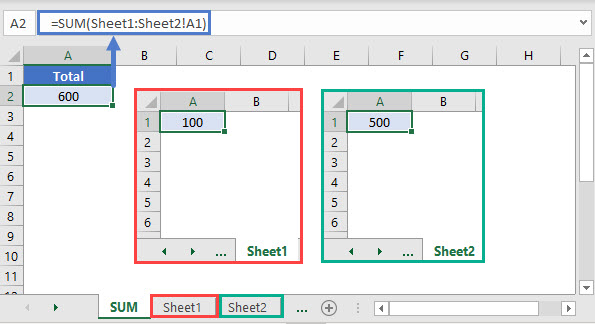However, this is not possible with the SUMIFS Function. Instead we must use a more complicated formula.

## Sum if Across Multiple Sheets

This example will sum the Number of Planned Deliveries for each Customer over multiple worksheets, each holding data relating to a different month, by using the SUMIFS, SUMPRODUCT, and INDIRECT Functions:

``=SUMPRODUCT(SUMIFS(INDIRECT("'"&F3:F6&"'!"&"D3:D7"),INDIRECT("'"&F3:F6&"'!"&"C3:C7"),H3))``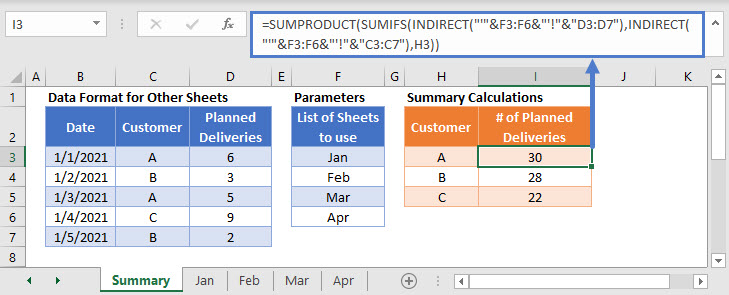Let’s walk through this formula.

### Step 1: Create a SUMIFS Formula for 1 Input Sheet Only:

We use the SUMIFS Function to sum the Number of Planned Deliveries by Customer for a single input data sheet:

``=SUMIFS(D3:D7,C3:C7,H3)``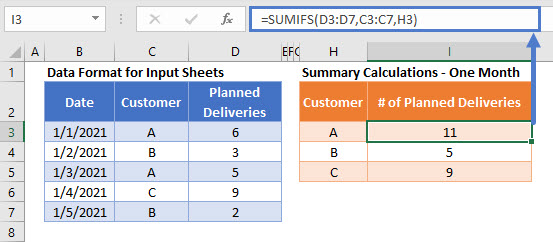### Step 2: Add a Sheet Reference to the Formula

We keep the formula result the same, but we specify that the input data is in the sheet called ‘Step 2’

``=SUMIFS('Step 2'!D3:D7,'Step 2'!C3:C7,H3)``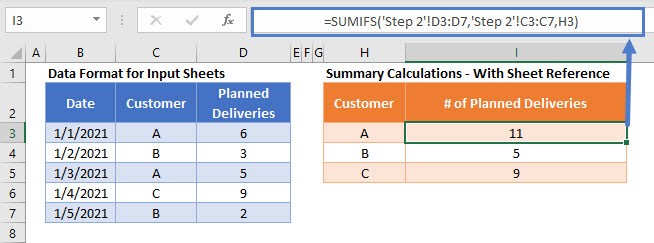### Step 3 : Nest Inside a SUMPRODUCT Function

To prepare the formula to perform SUMIFS calculations over multiple sheets and then to sum the results together, we add a SUMPRODUCT Function around the formula

``=SUMPRODUCT(SUMIFS('Step 3'!D3:D7,'Step 3'!C3:C7,H3))``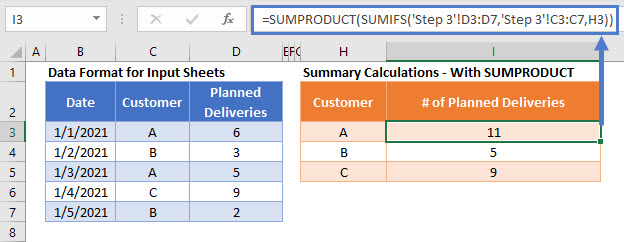Using the SUMIFS Function on one sheet yields a single value. Across multiple sheets, the SUMIFS function outputs an array of values (one for each worksheet). We use the SUMPRODUCT Function to total the values in this array.

### Step 4: Replace the Sheet Reference with a List of Sheet Names

We wish to replace the Sheet Name part of the formula with a data list containing the values: Jan, Feb, Mar, and Apr. This list is stored in the cells F3:F6.

The INDIRECT Function to ensures that the text list showing Sheet Names is treated as part of a valid cell reference in the SUMIFS Function.

``=SUMPRODUCT(SUMIFS(INDIRECT("'"&F3:F6&"'!"&"D3:D7"),INDIRECT("'"&F3:F6&"'!"&"C3:C7"),H3))``In this formula, the previously written range reference:

``'Step 3'!D3:D7``

Is replaced by:

``INDIRECT("'"&F3:F6&"'!"&"D3:D7")``

The quotation marks makes the formula difficult to read, so here it is shown with added spaces:

``INDIRECT ( " ' " & F3:F6 & " ' ! " & "D3:D7" )``

Using this way of referencing a list of cells also allows us to summarize data from multiple sheets which do not follow a numerical list style. A standard 3D reference would require the sheet names to be in the style: Input1, Input2, Input3, etc., but the example above allows you to use a list of any Sheet Names and to have them referenced in a separate cell.

### Easy Excel Automation## Locking Cell References

To make our formulas easier to read, we’ve shown the formulas without locked cell references:

``=SUMPRODUCT(SUMIFS(INDIRECT("'"&F3:F6&"'!"&"D3:D7"),INDIRECT("'"&F3:F6&"'!"&"C3:C7"),H3))``

But these formulas will not work properly when copy and pasted elsewhere in your file. Instead, you should use locked cell references like this:

``=SUMPRODUCT(SUMIFS(INDIRECT("'"&\$F\$3:\$F\$6&"'!"&"D3:D7"),INDIRECT("'"&\$F\$3:\$F\$6&"'!"&"C3:C7"),H3))``

## Sum If Across Multiple Sheets in Google Sheets

Using the INDIRECT Function to reference a list of sheets in a SUMPRODUCT and SUMIFS Function is not currently possible in Google Sheets.

``````=SUMIFS(Jan!D3:D7,Jan!C3:C7,H3)
+SUMIFS(Feb!D3:D7,Feb!C3:C7,H3)
+SUMIFS(Mar!D3:D7,Mar!C3:C7,H3)
+SUMIFS(Apr!D3:D7,Apr!C3:C7,H3)``````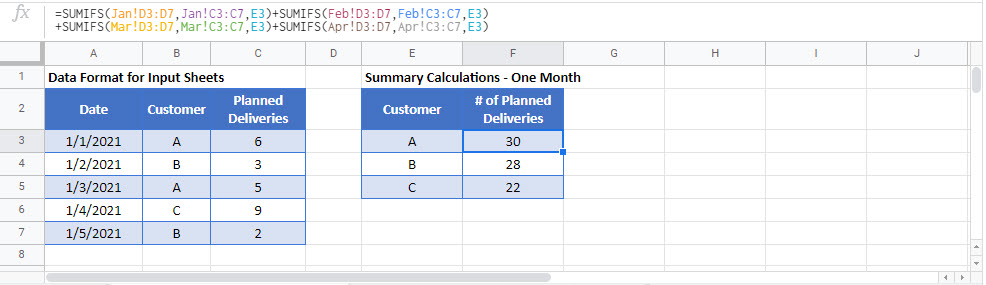### Excel Practice Worksheet

Practice Excel functions and formulas with our 100% free practice worksheets!